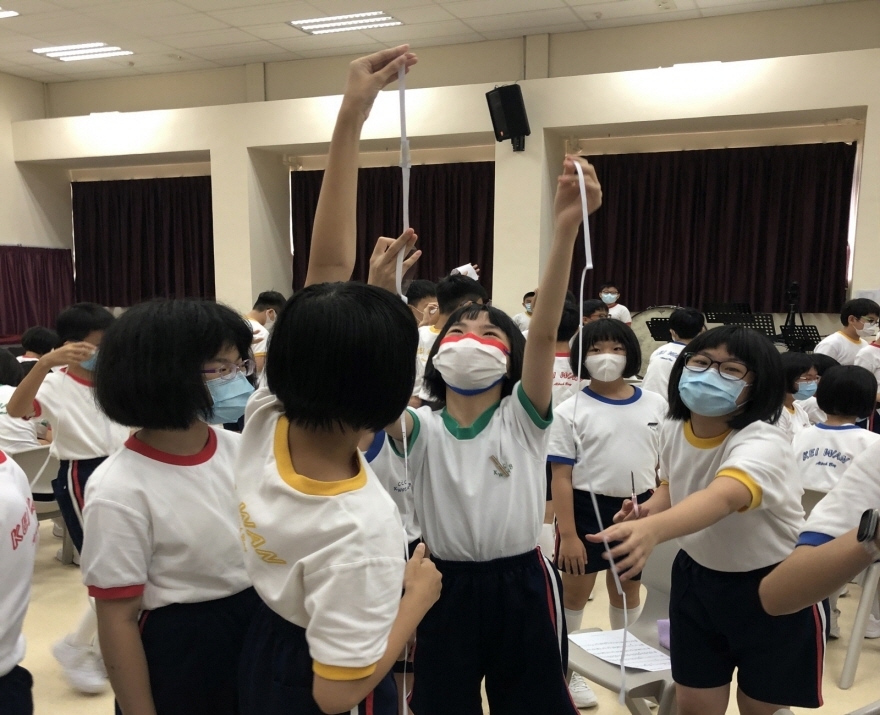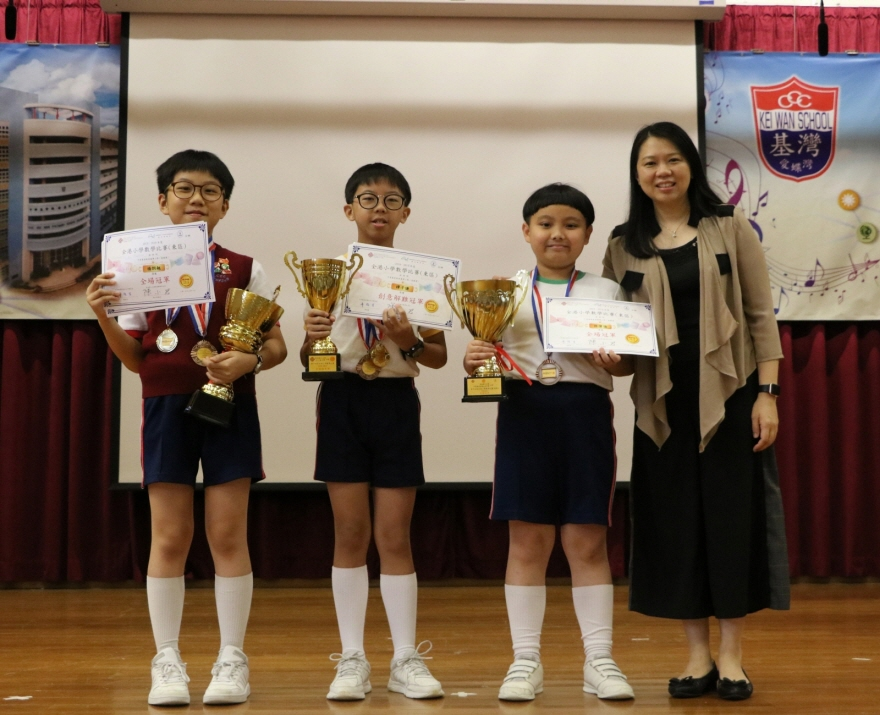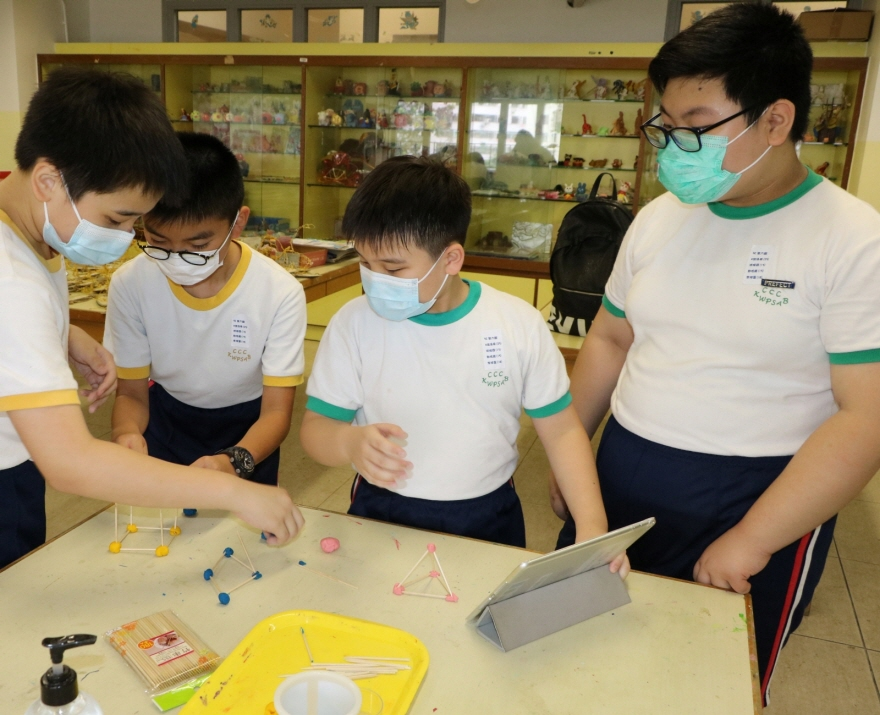Courses
MathematicsObjectives:

• To enhance students’ interest in learning Mathematics
• To understand basic mathematical concepts and acquire calculation skills
• To develop students’ abilities to explore, communicate, give reasons and solve problems, etc.
• To explore the patterns and structures of numbers and graphs
• To cultivate students to develop a positive attitude towards learning

School-based Curriculum

• School-based exercise books for Mathematics:

Objectives: To enhance students’ self-learning skills and encourage students to make self-improvement

• School-based worksheets for Mathematics:

Objectives: To improve students’ mathematical problem solving skills

• Training courses for Mathematical Olympiad:

Objectives: To develop students’ knowledge of higher Mathematics

Activities:

1. School activities: Mathematics Week (2 weeks), inter-class Speedy Maths competitions, P.1-3 inter-class and P.4-6 inter-house Mathematics competitions, Activities conducted at Maths corner, Mathematics Day
2. Competitions outside school: Hong Kong Mathematical Olympiad, ‘Huaxiabei’ National Mathematics Olympiad Competition, Hong Kong & Macau Mathematical Olympiad Open Contest ‘HKMO’, Sudoku Competition for Kids under 12 World Class Tests, Hong Kong Primary Mathematics Challenge (Eastern District), Hong Kong Mathematics Creative Problem Solving Competition (Primary Schools), Rummikub Competition
﻿